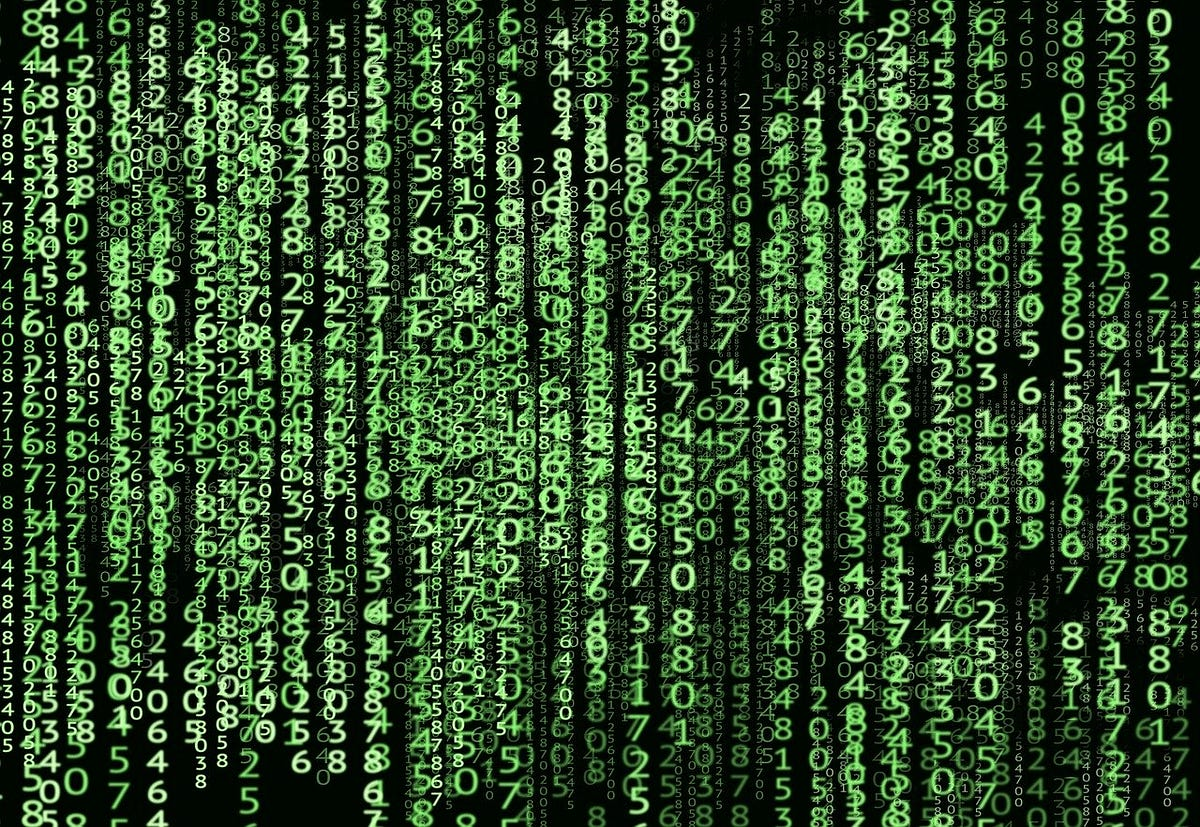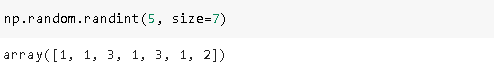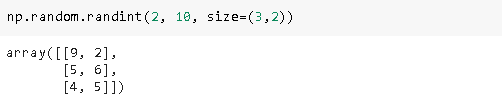# 20 NumPy Operations That Every Data Scientist Should KnowNumPy forms the basis of many Python libraries in the data science domain. Everything about data science starts with data and it comes in various formats.

Everything about data science starts with data and it comes in various formats. Numbers, images, texts, x-rays, sound, and video recordings are just some examples of data sources. Whatever the format data comes in, it needs to be converted to an array of numbers to be analyzed. Hence, it is crucial to effectively store and modify arrays of numbers in data science.

*NumPy *(Numerical Python) is a scientific computing package that provides numerous ways to create and operate on arrays of numbers. It forms the basis of many widely used Python libraries related to data science such as Pandas and Matplotlib.

In this post, I will go over 20 commonly used operations on NumPy arrays. These operations can be grouped under 4 main categories:

• Creating arrays
• Manipulating arrays
• Combining arrays
• Linear algebra with arrays

We first need to import NumPy:

``import numpy as np``

## Creating arrays

1. Random integers in a specific rangeThe first parameter determines the upper bound of the range. The lower bound is 0 by default but we can also specify it. The *size *parameter is used to specify the size, as expected.We created a 3x2 array of integers between 2 and 10.

## Most popular Data Science and Machine Learning courses — July 2020

Most popular Data Science and Machine Learning courses — August 2020. This list was last updated in August 2020 — and will be updated regularly so as to keep it relevant

## Artificial Intelligence (AI) vs Machine Learning vs Deep Learning vs Data Science

Artificial Intelligence (AI) vs Machine Learning vs Deep Learning vs Data Science: Artificial intelligence is a field where set of techniques are used to make computers as smart as humans. Machine learning is a sub domain of artificial intelligence where set of statistical and neural network based algorithms are used for training a computer in doing a smart task. Deep learning is all about neural networks. Deep learning is considered to be a sub field of machine learning. Pytorch and Tensorflow are two popular frameworks that can be used in doing deep learning.

## Artificial Intelligence vs Machine Learning vs Data Science

Artificial Intelligence, Machine Learning, and Data Science are amongst a few terms that have become extremely popular amongst professionals in almost all the fields.

## Pipelines in Machine Learning | Data Science | Machine Learning | Python

Machine Learning Pipelines performs a complete workflow with an ordered sequence of the process involved in a Machine Learning task. The Pipelines can also

## Data Science Projects | Data Science | Machine Learning | Python

Practice your skills in Data Science with Python, by learning and then trying all these hands-on, interactive projects, that I have posted for you.